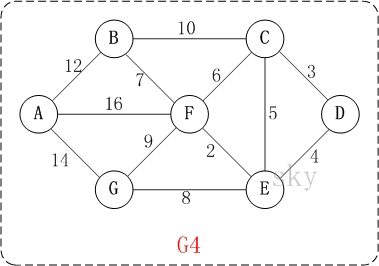# Dijkstra算法图解此时，S={D(0)}, U={A(∞),B(∞),C(3),E(4),F(∞),G(∞)}。     注:C(3)表示C到起点D的距离是3。

上一步操作之后，U中顶点C到起点D的距离最短；因此，将C加入到S中，同时更新U中顶点的距离。以顶点F为例，之前F到D的距离为∞；但是将C加入到S之后，F到D的距离为9=(F,C)+(C,D)。
此时，S={D(0),C(3)}, U={A(∞),B(23),E(4),F(9),G(∞)}。

上一步操作之后，U中顶点E到起点D的距离最短；因此，将E加入到S中，同时更新U中顶点的距离。还是以顶点F为例，之前F到D的距离为9；但是将E加入到S之后，F到D的距离为6=(F,E)+(E,D)。
此时，S={D(0),C(3),E(4)}, U={A(∞),B(23),F(6),G(12)}。

此时，S={D(0),C(3),E(4),F(6)}, U={A(22),B(13),G(12)}。

此时，S={D(0),C(3),E(4),F(6),G(12)}, U={A(22),B(13)}。

此时，S={D(0),C(3),E(4),F(6),G(12),B(13)}, U={A(22)}。

此时，S={D(0),C(3),E(4),F(6),G(12),B(13),A(22)}。

1.111 says: